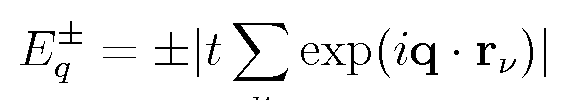Graphene Band Structure

R. B. Laughlin May 19, 2008

(Submitted as coursework for Physics 373, Stanford University, Spring 2008)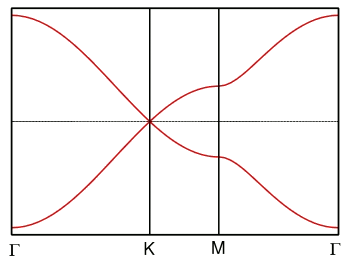Fig. 1: This is a prototype figure. Note that clicking on this figure brings up the high-resolution original. The computer code that generated these bands is available here. I plotted using gnuplot. The plot file is shown here. I used gimp to shrink the figure, after first fattening its lines. I also shrank the labels at the bottom less. I did this by shrinking a second copy of the figure, excising its labels and pasting them in.

This is a report template that shows the band structure of graphene. The equation plotted is the last one below. In this equation the three vectors rν, given by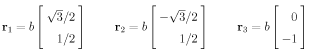are the translation vectors taking one carbon to its three near neighbors. Note that there are two inequivalent atoms in the unit cell. The translation vectors taking this second atom to its near neighbors are thus - rν. The bravais lattice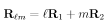where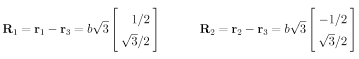is the test of all translations from an atom to any equivalent atom. To this bravais lattice there is a corresponding reciprocal lattice defined bywhere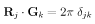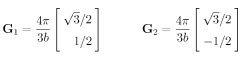The crystal momentum q of an electron is defined only modulo addition of any reciprocal lattice vectors. We thus need consider the states only in the first brillouin zone, the set of all crystal momenta q closer to the origin than to any other reciprocal lattice vector. The brillouin zone in this case is a hexagon. We cannot plot the bands everywhere, so we just plot them on a representative "tour" of the brillouin zone. The choice of tour is somewhat arbitrary. You do what you think makes the situation clear. The first leg of my tour is a straight line from the zone center (Γ) to the hexagon vertex (K), from there to the mid point of the face (M), and from there back to the origin (Γ). The special points are given by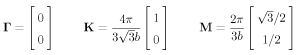The band energies are given by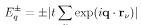The technology I use for equations is to make a LaTeX source, output to PostScript using dvips and then using gimp to chop out the equation from the PostScript and convert it to .gif format. Under my version of gimp there is a choice of resolution when importing PostScript. I choose 300 dpi because my version of dvips prints at 300 dpi. The graphic thus created is very crisp - pure black-and-white, no gray. It is, however, at inappropriate resolution for Internet use. You can, if you wish, put a scaling command into your "img" entry for the equation, but I have chosen just to shrink the equation image to 1/4 its original linear dimension. This factor of 1/4 comes from screen resolution, which is typically 72 dpi. You must be careful in shrinking to minimize resolution degradation. I have found by experiment that this is best done by the 3-stop process (1) convert image to grayscale, (2) scale image and (3) re-convert to indexed with 256 colors. It is important to store equations in .gif format rather than .jpg because the latter tends to put speckle in the whitespace, due to the compression algorithm. An example of an original, uncompressed is shown below. The original LaTeX source for these equations is available here.